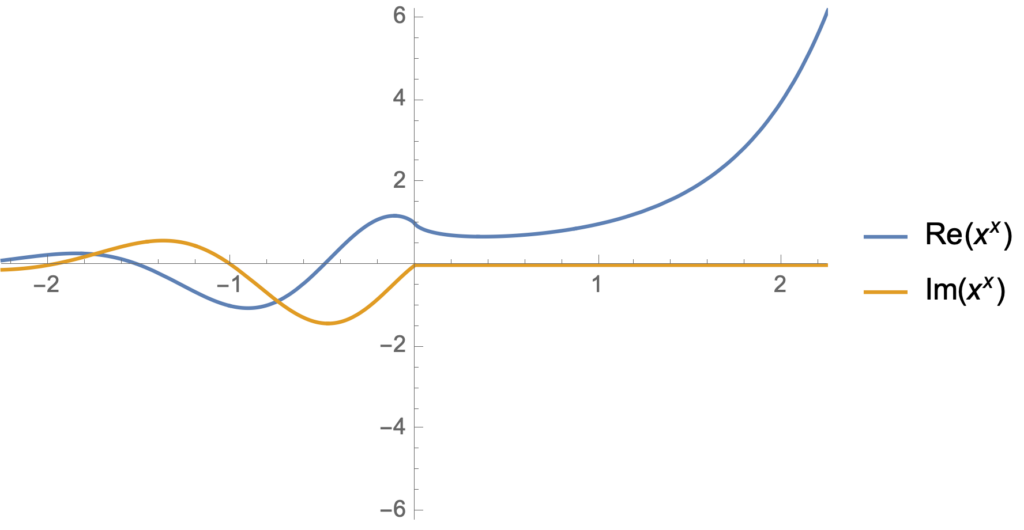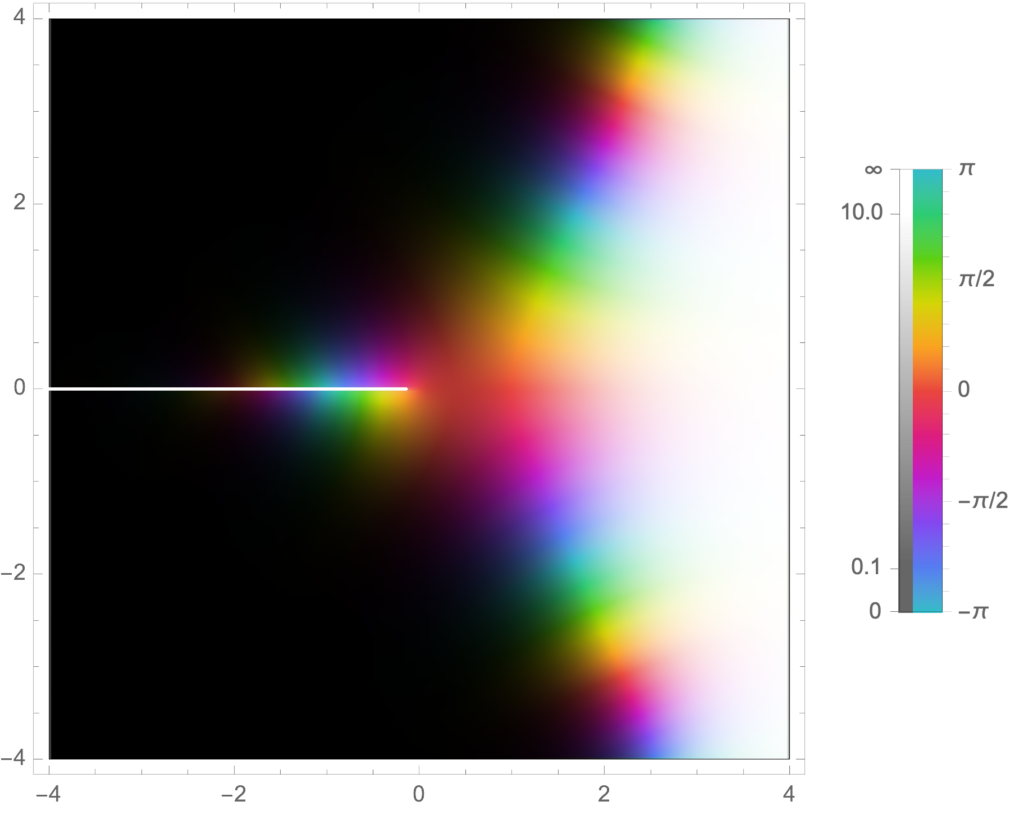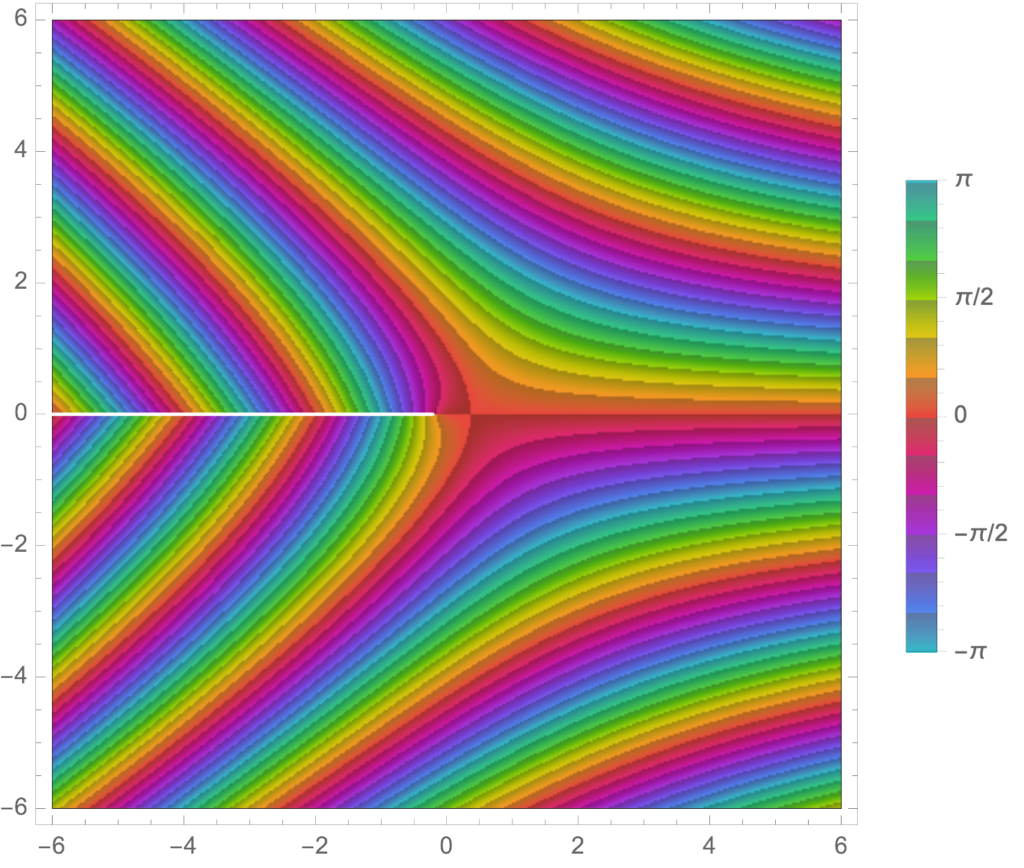## Exploring 0^0, x^x, and z^z.

My Youtube home page knows that I’m geeky enough to watch math videos.  Today it suggested Eddie Woo’s video about $$0^0$$.

Mr Woo, who has great enthusiasm, and must be an awesome teacher to have in person.  He reminds his class about the exponent laws, which allow for an interpretation that $$0^0$$ would be equal to 1.  He points out that $$0^n = 0$$ for any positive integer, which admits a second contradictory value for $$0^0$$, if this was true for $$n=0$$ too.

When reviewing the exponent laws Woo points out that the exponent law for subtraction $$a^{n-n}$$ requires $$a$$ to be non-zero.  Given that restriction, we really ought to have no expectation that $$0^{n-n} = 1$$.

To attempt to determine a reasonable value for this question, resolving the two contradictory possibilities, neither of which we actually have any reason to assume are valid possibilities, he asks the class to perform a proof by calculator, computing a limit table for $$x \rightarrow 0+$$. I stopped at that point and tried it by myself, constructing such a table in Mathematica. Here is what I used

griddisp[labelc1_, labelc2_, f_, values_] := Grid[({
({{labelc1}, values}) // Flatten,
({ {labelc2}, f[#] & /@ values} ) // Flatten
}) // Transpose,
Frame -> All]
decimalFractions[n_] := ((10^(-#)) & /@ Range[n])
With[{m = 10}, griddisp[x, x^x, #^# &, N[decimalFractions[m], 10]]]
With[{m = 10}, griddisp[x, x^x, #^# &, -N[decimalFractions[m], 10]]]


Observe that I calculated the limits from both above and below. The results areand for the negative limitSure enough, from both below and above, we see numerically that $$\lim_{\epsilon\rightarrow 0} \epsilon^\epsilon = 1$$, as if the exponent law argument for $$0^0 = 1$$ was actually valid.  We see that this limit appears to be valid despite the fact that $$x^x$$ can be complex valued — that is ignoring the fact that a rigorous limit argument should be valid for any path neighbourhood of $$x = 0$$ and not just along two specific (real valued) paths.

Let’s get a better idea where the imaginary component of $$(-x)^{-x}$$ comes from.  To do so, consider $$f(z) = z^z$$ for complex values of $$z$$ where $$z = r e^{i \theta}$$. The logarithm of such a beast is

\begin{equation}\label{eqn:xtox:20}
\begin{aligned}
\ln z^z
&= z \ln \lr{ r e^{i\theta} } \\
&= z \ln r + i \theta z \\
&= e^{i\theta} \ln r^r + i \theta z \\
&= \lr{ \cos\theta + i \sin\theta } \ln r^r + i r \theta \lr{ \cos\theta + i \sin\theta } \\
&= \cos\theta \ln r^r – r \theta \sin\theta
+ i r \lr{ \sin\theta \ln r + \theta \cos\theta },
\end{aligned}
\end{equation}
so
\begin{equation}\label{eqn:xtox:40}
z^z =
e^{ r \lr{ \cos\theta \ln r – \theta \sin\theta}} \times
e^{i r \lr{ \sin\theta \ln r + \theta \cos\theta }}.
\end{equation}
In particular, picking the $$\theta = \pi$$ branch, we have, for any $$x > 0$$
\begin{equation}\label{eqn:xtox:60}
(-x)^{-x} = e^{-x \ln x – i x \pi } = \frac{e^{ – i x \pi }}{x^x}.
\end{equation}

Let’s get some visual appreciation for this interesting $$z^z$$ beastie, first plotting it for real values of $$z$$


Manipulate[
Plot[ {Re[x^x], Im[x^x]}, {x, -r, r}
, PlotRange -> {{-r, r}, {-r^r, r^r}}
, PlotLegends -> {Re[x^x], Im[x^x]}
], {{r, 2.25}, 0.0000001, 10}]From this display, we see that the imaginary part of $$x^x$$ is zero for integer values of $$x$$.  That’s easy enough to verify explicitly: $$(-1)^{-1} = -1, (-2)^{-2} = 1/4, (-3)^{-3} = -1/27, \cdots$$.

The newest version of Mathematica has a few nice new complex number visualization options.  Here’s two that I found illuminating, an absolute value plot that highlights the poles and zeros, also showing some of the phase action:

Manipulate[
ComplexPlot[ x^x, {x, s (-1 – I), s (1 + I)},
PlotLegends -> Automatic, ColorFunction -> "GlobalAbs"], {{s, 4},
0.00001, 10}]We see the branch cut nicely, the tendency to zero in the left half plane, as well as some of the phase periodicity in the regions that are in the intermediate regions between the zeros and the poles.  We can also plot just the phase, which shows its interesting periodic nature


Manipulate[
ComplexPlot[ x^x, {x, s (-1 – I), s (1 + I)},
PlotLegends -> Automatic, ColorFunction -> "CyclicArg"], {{s, 6},
0.00001, 10}]I’d like to take the time to play with some of the other ComplexPlot ColorFunction options, which appears to be a powerful and flexible visualization tool.

## Line charge field and potential.

When computing the most general solution of the electrostatic potential in a plane, Jackson  mentions that $$-2 \lambda_0 \ln \rho$$ is the well known potential for an infinite line charge (up to the unit specific factor). Checking that statement, since I didn’t recall what that potential was offhand, I encountered some inconsistencies and non-convergent integrals, and thought it was worthwhile to explore those a bit more carefully. This will be done here.

### Using Gauss’s law.

For an infinite length line charge, we can find the radial field contribution using Gauss’s law, imagining a cylinder of length $$\Delta l$$ of radius $$\rho$$ surrounding this charge with the midpoint at the origin. Ignoring any non-radial field contribution, we have

\begin{equation}\label{eqn:lineCharge:20}
\int_{-\Delta l/2}^{\Delta l/2} \ncap \cdot \BE (2 \pi \rho) dl = \frac{\lambda_0}{\epsilon_0} \Delta l,
\end{equation}

or

\begin{equation}\label{eqn:lineCharge:40}
\BE = \frac{\lambda_0}{2 \pi \epsilon_0} \frac{\rhocap}{\rho}.
\end{equation}

Since

\begin{equation}\label{eqn:lineCharge:60}
\end{equation}

this means that the potential is

\begin{equation}\label{eqn:lineCharge:80}
\phi = -\frac{2 \lambda_0}{4 \pi \epsilon_0} \ln \rho.
\end{equation}

### Finite line charge potential.

Let’s try both these calculations for a finite charge distribution. Gauss’s law looses its usefulness, but we can evaluate the integrals directly. For the electric field

\begin{equation}\label{eqn:lineCharge:100}
\BE
= \frac{\lambda_0}{4 \pi \epsilon_0} \int \frac{(\Bx – \Bx’)}{\Abs{\Bx – \Bx’}^3} dl’.
\end{equation}

Using cylindrical coordinates with the field point $$\Bx = \rho \rhocap$$ for convience, the charge point $$\Bx’ = z’ \zcap$$, and a the charge distributed over $$[a,b]$$ this is

\begin{equation}\label{eqn:lineCharge:120}
\BE
= \frac{\lambda_0}{4 \pi \epsilon_0} \int_a^b \frac{(\rho \rhocap – z’ \zcap)}{\lr{\rho^2 + (z’)^2}^{3/2}} dz’.
\end{equation}

When the charge is uniformly distributed around the origin $$[a,b] = b[-1,1]$$ the $$\zcap$$ component of this field is killed because the integrand is odd. This justifies ignoring such contributions in the Gaussing cylinder analysis above. The general solution to this integral is found to be

\begin{equation}\label{eqn:lineCharge:140}
\BE
=
\frac{\lambda_0}{4 \pi \epsilon_0}
\evalrange{
\lr{
\frac{z’ \rhocap }{\rho \sqrt{ \rho^2 + (z’)^2 } }
+\frac{\zcap}{ \sqrt{ \rho^2 + (z’)^2 } }
}
}{a}{b},
\end{equation}

or
\begin{equation}\label{eqn:lineCharge:240}
\boxed{
\BE
=
\frac{\lambda_0}{4 \pi \epsilon_0}
\lr{
\frac{\rhocap }{\rho}
\lr{
\frac{b}{\sqrt{ \rho^2 + b^2 } }
-\frac{a}{\sqrt{ \rho^2 + a^2 } }
}
+ \zcap
\lr{
\frac{1}{ \sqrt{ \rho^2 + b^2 } }
-\frac{1}{ \sqrt{ \rho^2 + a^2 } }
}
}.
}
\end{equation}

When $$b = -a = \Delta l/2$$, this reduces to

\begin{equation}\label{eqn:lineCharge:160}
\BE
=
\frac{\lambda_0}{4 \pi \epsilon_0}
\frac{\rhocap }{\rho}
\frac{\Delta l}{\sqrt{ \rho^2 + (\Delta l/2)^2 } },
\end{equation}

which further reduces to \ref{eqn:lineCharge:40} when $$\Delta l \gg \rho$$.

### Finite line charge potential. Wrong but illuminating.

Again, putting the field point at $$z’ = 0$$, we have

\begin{equation}\label{eqn:lineCharge:180}
\phi(\rho)
= \frac{\lambda_0}{4 \pi \epsilon_0} \int_a^b \frac{dz’}{\lr{\rho^2 + (z’)^2}^{1/2}},
\end{equation}

which integrates to
\begin{equation}\label{eqn:lineCharge:260}
\phi(\rho)
= \frac{\lambda_0}{4 \pi \epsilon_0 }
\ln \frac{ b + \sqrt{ \rho^2 + b^2 }}{ a + \sqrt{\rho^2 + a^2}}.
\end{equation}

With $$b = -a = \Delta l/2$$, this approaches

\begin{equation}\label{eqn:lineCharge:200}
\phi
\approx
\frac{\lambda_0}{4 \pi \epsilon_0 }
\ln \frac{ (\Delta l/2) }{ \rho^2/2\Abs{\Delta l/2}}
=
\frac{-2 \lambda_0}{4 \pi \epsilon_0 } \ln \rho
+
\frac{\lambda_0}{4 \pi \epsilon_0 }
\ln \lr{ (\Delta l)^2/2 }.
\end{equation}

Before $$\Delta l$$ is allowed to tend to infinity, this is identical (up to a difference in the reference potential) to \ref{eqn:lineCharge:80} found using Gauss’s law. It is, strictly speaking, singular when $$\Delta l \rightarrow \infty$$, so it does not seem right to infinity as a reference point for the potential.

There’s another weird thing about this result. Since this has no $$z$$ dependence, it is not obvious how we would recover the non-radial portion of the electric field from this potential using $$\BE = -\spacegrad \phi$$? Let’s calculate the elecric field from \ref{eqn:lineCharge:180} explicitly

\begin{equation}\label{eqn:lineCharge:220}
\begin{aligned}
\BE
&=
-\frac{\lambda_0}{4 \pi \epsilon_0}
\ln \frac{ b + \sqrt{ \rho^2 + b^2 }}{ a + \sqrt{\rho^2 + a^2}} \\
&=
-\frac{\lambda_0 \rhocap}{4 \pi \epsilon_0 }
\PD{\rho}{}
\ln \frac{ b + \sqrt{ \rho^2 + b^2 }}{ a + \sqrt{\rho^2 + a^2}} \\
&=
-\frac{\lambda_0 \rhocap}{4 \pi \epsilon_0}
\lr{
\inv{ b + \sqrt{ \rho^2 + b^2 }} \frac{ \rho }{\sqrt{ \rho^2 + b^2 }}
-\inv{ a + \sqrt{ \rho^2 + a^2 }} \frac{ \rho }{\sqrt{ \rho^2 + a^2 }}
} \\
&=
-\frac{\lambda_0 \rhocap}{4 \pi \epsilon_0 \rho}
\lr{
\frac{ -b + \sqrt{ \rho^2 + b^2 }}{\sqrt{ \rho^2 + b^2 }}
-\frac{ -a + \sqrt{ \rho^2 + a^2 }}{\sqrt{ \rho^2 + a^2 }}
} \\
&=
\frac{\lambda_0 \rhocap}{4 \pi \epsilon_0 \rho}
\lr{
\frac{ b }{\sqrt{ \rho^2 + b^2 }}
-\frac{ a }{\sqrt{ \rho^2 + a^2 }}
}.
\end{aligned}
\end{equation}

This recovers the radial component of the field from \ref{eqn:lineCharge:240}, but where did the $$\zcap$$ component go? The required potential appears to be

\begin{equation}\label{eqn:lineCharge:340}
\phi(\rho, z)
=
\frac{\lambda_0}{4 \pi \epsilon_0 }
\ln \frac{ b + \sqrt{ \rho^2 + b^2 }}{ a + \sqrt{\rho^2 + a^2}}

\frac{z \lambda_0}{4 \pi \epsilon_0 }
\lr{ \frac{1}{\sqrt{\rho^2 + b^2}}
-\frac{1}{\sqrt{\rho^2 + a^2}}
}.
\end{equation}

When computing the electric field $$\BE(\rho, \theta, z)$$, it was convienent to pick the coordinate system so that $$z = 0$$. Doing this with the potential gives the wrong answers. The reason for this appears to be that this kills the potential term that is linear in $$z$$ before taking its gradient, and we need that term to have the $$\zcap$$ field component that is expected for a charge distribution that is non-symmetric about the origin on the z-axis!

### Finite line charge potential. Take II.

Let the point at which the potential is evaluated be

\begin{equation}\label{eqn:lineCharge:360}
\Bx = \rho \rhocap + z \zcap,
\end{equation}

and the charge point be
\begin{equation}\label{eqn:lineCharge:380}
\Bx’ = z’ \zcap.
\end{equation}

This gives

\begin{equation}\label{eqn:lineCharge:400}
\begin{aligned}
\phi(\rho, z)
&= \frac{\lambda_0}{4\pi \epsilon_0} \int_a^b \frac{dz’}{\Abs{\rho^2 + (z – z’)^2 }} \\
&= \frac{\lambda_0}{4\pi \epsilon_0} \int_{a-z}^{b-z} \frac{du}{ \Abs{\rho^2 + u^2} } \\
&= \frac{\lambda_0}{4\pi \epsilon_0}
\evalrange{\ln \lr{ u + \sqrt{ \rho^2 + u^2 }}}{b-z}{a-z} \\
&=
\frac{\lambda_0}{4\pi \epsilon_0}
\ln \frac
{ b-z + \sqrt{ \rho^2 + (b-z)^2 }}
{ a-z + \sqrt{ \rho^2 + (a-z)^2 }}.
\end{aligned}
\end{equation}

The limit of this potential $$a = -\Delta/2 \rightarrow -\infty, b = \Delta/2 \rightarrow \infty$$ doesn’t exist in any strict sense. If we are cavilier about the limits, as in \ref{eqn:lineCharge:200}, this can be evaluated as

\begin{equation}\label{eqn:lineCharge:n}
\phi \approx
\frac{\lambda_0}{4\pi \epsilon_0} \lr{ -2 \ln \rho + \textrm{constant} }.
\end{equation}

however, the constant ($$\ln \Delta^2/2$$) is infinite, so there isn’t really a good justification for using that constant as the potential reference point directly.

It seems that the “right” way to calculate the potential for the infinite distribution, is to

• Calculate the field from the potential.
• Take the PV limit of that field with the charge distribution extending to infinity.
• Compute the corresponding potential from this limiting value of the field.

Doing that doesn’t blow up. That field calculation, for the finite case, should include a $$\zcap$$ component. To verify, let’s take the respective derivatives

\begin{equation}\label{eqn:lineCharge:420}
\begin{aligned}
-\PD{z}{} \phi
&=
-\frac{\lambda_0}{4\pi \epsilon_0}
\lr{
\frac{ -1 + \frac{z – b}{\sqrt{ \rho^2 + (b-z)^2 }} }{
b-z + \sqrt{ \rho^2 + (b-z)^2 }
}

\frac{ -1 + \frac{z – a}{\sqrt{ \rho^2 + (a-z)^2 }} }{
a-z + \sqrt{ \rho^2 + (a-z)^2 }
}
} \\
&=
\frac{\lambda_0}{4\pi \epsilon_0}
\lr{
\frac{ 1 + \frac{b – z}{\sqrt{ \rho^2 + (b-z)^2 }} }{
b-z + \sqrt{ \rho^2 + (b-z)^2 }
}

\frac{ 1 + \frac{a – z}{\sqrt{ \rho^2 + (a-z)^2 }} }{
a-z + \sqrt{ \rho^2 + (a-z)^2 }
}
} \\
&=
\frac{\lambda_0}{4\pi \epsilon_0}
\lr{
\inv{\sqrt{ \rho^2 + (b-z)^2 }}
-\inv{\sqrt{ \rho^2 + (a-z)^2 }}
},
\end{aligned}
\end{equation}

and

\begin{equation}\label{eqn:lineCharge:440}
\begin{aligned}
-\PD{\rho}{} \phi
&=
-\frac{\lambda_0}{4\pi \epsilon_0}
\lr{
\frac{ \frac{\rho}{\sqrt{ \rho^2 + (b-z)^2 }} }{
b-z + \sqrt{ \rho^2 + (b-z)^2 }
}

\frac{ \frac{\rho}{\sqrt{ \rho^2 + (a-z)^2 }} }{
a-z + \sqrt{ \rho^2 + (a-z)^2 }
}
} \\
&=
-\frac{\lambda_0}{4\pi \epsilon_0}
\lr{
\frac{\rho \lr{
-(b-z) + \sqrt{ \rho^2 + (b-z)^2 }
}}{ \rho^2 \sqrt{ \rho^2 + (b-z)^2 } }

\frac{\rho \lr{
-(a-z) + \sqrt{ \rho^2 + (a-z)^2 }
}}{ \rho^2 \sqrt{ \rho^2 + (a-z)^2 } }
} \\
&=
\frac{\lambda_0}{4\pi \epsilon_0 \rho}
\lr{
\frac{b-z}{\sqrt{ \rho^2 + (b-z)^2 }}
-\frac{a-z}{\sqrt{ \rho^2 + (a-z)^2 }}
}
.
\end{aligned}
\end{equation}

Putting the pieces together, the electric field is
\begin{equation}\label{eqn:lineCharge:460}
\BE =
\frac{\lambda_0}{4\pi \epsilon_0}
\lr{
\frac{\rhocap}{\rho} \lr{
\frac{b-z}{\sqrt{ \rho^2 + (b-z)^2 }}
-\frac{a-z}{\sqrt{ \rho^2 + (a-z)^2 }}
}
+
\zcap \lr{
\inv{\sqrt{ \rho^2 + (b-z)^2 }}
-\inv{\sqrt{ \rho^2 + (a-z)^2 }}
}
}.
\end{equation}

For has a PV limit of \ref{eqn:lineCharge:40} at $$z = 0$$, and also for the finite case, has the $$\zcap$$ field component that was obtained when the field was obtained by direct integration.

### Conclusions

• We have to evaluate the potential at all points in space, not just on the axis that we evaluate the field on (should we choose to do so).
• In this case, we found that it was not directly meaningful to take the limit of a potential distribution. We can, however, compute the field from a potential for a finite charge distribution,
take the limit of that field, and then calculate the corresponding potential for the infinite distribution.

Is there a more robust mechanism that can be used to directly calculate the potential for an infinite charge distribution, instead of calculating the potential from the field of such an infinite distribution?

I think that were things go wrong is that the integral of \ref{eqn:lineCharge:180} does not apply to charge distributions that are not finite on the infinite range $$z \in [-\infty, \infty]$$. That solution was obtained by utilizing an all-space Green’s function, and the boundary term in that Green’s analysis was assumed to tend to zero. That isn’t the case when the charge distribution is $$\lambda_0 \delta( z )$$.

# References

 JD Jackson. Classical Electrodynamics. John Wiley and Sons, 2nd edition, 1975.